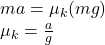Question

A block with mass m is pulled horizontally with a force F_pull leading to an acceleration a along a rough, flat surface.
Find the coefficient of kinetic friction between the block and the surface.

1.thuthuy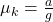Explanation:

The force of kinetic friction on the block is defined as: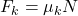Where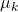is the coefficient of kinetic friction between the block and the surface and N is the normal force, which is always perpendicular to the surface that the object contacts. So, according to the free body diagram of the block, we have: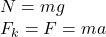Replacing this in the first equation and solving for: